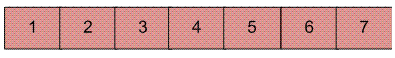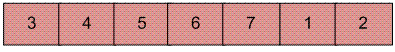Python Program for Reversal algorithm for array rotation

• Difficulty Level : Medium
• Last Updated : 29 Apr, 2020

Write a function rotate(arr[], d, n) that rotates arr[] of size n by d elements.

Example :

Input :  arr[] = [1, 2, 3, 4, 5, 6, 7]
d = 2
Output : arr[] = [3, 4, 5, 6, 7, 1, 2]Rotation of the above array by 2 will make arrayRecommended: Please solve it on “PRACTICE ” first, before moving on to the solution.

 # Python program for reversal algorithm of array rotation  # Function to reverse arr[] from index start to enddef rverseArray(arr, start, end):    while (start < end):        temp = arr[start]        arr[start] = arr[end]        arr[end] = temp        start += 1        end = end-1  # Function to left rotate arr[] of size n by ddef leftRotate(arr, d):    n = len(arr)    rverseArray(arr, 0, d-1)    rverseArray(arr, d, n-1)    rverseArray(arr, 0, n-1)  # Function to print an arraydef printArray(arr):    for i in range(0, len(arr)):        print (arr[i])  # Driver function to test above functionsarr = [1, 2, 3, 4, 5, 6, 7]leftRotate(arr, 2) # Rotate array by 2printArray(arr)  # This code is contributed by Devesh Agrawal

Output :

3 4 5 6 7 1 2

Time Complexity : O(n)

Please refer complete article on Reversal algorithm for array rotation for more details!

My Personal Notes arrow_drop_up